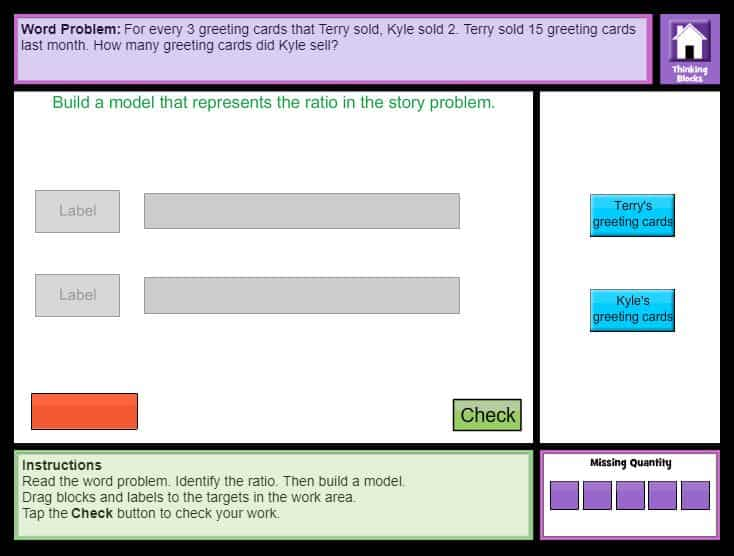# Interactive Problem Solving (6th Grade)

Thinking Blocks - Model and solve ratio and proportion word problems with Thinking Blocks (6th Grade).

#### Online Word Problems with Ratio and ProportionCCSS.MATH.CONTENT.6.RP.A.3
Use ratio and rate reasoning to solve real-world and mathematical problems, e.g., by reasoning about tables of equivalent ratios, tape diagrams, double number line diagrams, or equations.